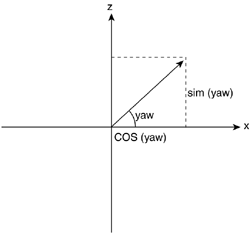# First-Person Shooters

The first camera approach we will explore is the first-person camera used in many first-person shooter titles, such as Quake. It is a camera defined by at least four degrees of freedom (X,Y,Z and yaw), with pitch sometimes added to the mix. As far as control goes, the camera uses the left and right cursor to rotate, and up and down are used to advance or move backward in the direction we are facing. A side movement called strafing is sometimes present, allowing us to advance sideways. Strafing is useful in dangerous corners.

The math for such a camera is not very complex. To begin with, here is the code (using an abstract input controller) for the left and right cursors:

` yaw+=ROTSPEED*elapsed*(input.right-input.left); `

In this line of code, input.left and input.right return 1 if the specified cursor is pressed, and 0 otherwise. The parenthetical expression will return 1 if we are pressing left, 0 if both (or none) are pressed, and 1 if right is pressed. The result of this parenthetical expression will act as a modifier to the first part of the expression. Basic physics tells us that

` Velocity=Space/Time `

Then, solving for Space we get

` Space=Velocity*Time `

which is fundamentally what we are doing here (albeit in an angular fashion) multiplying a constant ROTSPEED (which will determine how fast our character can turn) by the elapsed time; that is, the time it takes to complete a full game loop. By using this formula, we get nice, device-independent rotation speeds. The character will rotate at the same speed on different computers.

Now we need the code that handles the up and down cursors. It follows the same strategy:

` int dz=(input.up-input.down); playerpos.x= SPEED*elapsed*dz*cos(yaw); playerpos.z= SPEED*elapsed*dz*sin(yaw); `

In the preceding code, dz is just the same idea we used before to control which keys are pressed. The meat lies in the next two lines. As we did earlier, we'll use SPEED*elapsed to obtain device independence. To understand the next section (dz*cos(yaw)), a small diagram and some trigonometry is required. See Figure 16.1.

##### Figure 16.1. Trigonometrical sphere, FPS camera.Remember that the cosine and sine functions relate the angle in a right triangle to the length of its sides. In our case, we have the angle (the yaw), and we need to compute the length of the side contiguous to it (which will become the increment in x) and opposite to it (which will be z). We assume the hypotenuse to be 1 and scale by SPEED*elapsed*dz to reach the end result.

Strafing is just a variant of the advance controller just shown. The trick is that a strafe move is performed by advancing sideways that is, with a 90º increment with respect to regular movement. Here is the source code:

` int dstrafe=(input.straferight-input.strafeleft); playerpos.x= STRAFESPEED*elapsed*dstrafe*cos(yaw+3.1416/2); playerpos.z= STRAFESPEED*elapsed*dstrafe*sin(yaw+3.1416/2); `Core Techniques and Algorithms in Game Programming2003
ISBN: N/A
EAN: N/A
Year: 2004
Pages: 261